Aptitude & Reasoning

NTSE MAT Sample Paper 1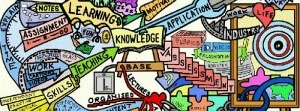This NTSE MAT Sample paper contains 90 questions as per pattern of CBSE NTSE exam. Answers are given at the end of questions.

Question: 1.12.91 is the first Sunday. Which is the fourth Tuesday of December 91 ?
(a) 17.12.91
(b) 24.12.91
(c) 26.12.91
(d) 31.12.91
Ans: (b)

Question: If February 1, 1996 is Wednesday, what day is March 3, 1996 ?
(a) Monday
(b) Sunday
(c) Saturday
(d) Friday
Ans. (c)

Direction for next 4 questions : In column I below, some words are given. In column II, their codes are given but they are not arranged in the same order in which they are in column I. Study the letters in both the columns and find out the code to the letter given in each of the following questions, from among the given alternatives.

 Column I Column II SOUND abi ADDRESS cjmv CRUX ikmop NET ij ktv CRONY jkgotv CROWDY blooppv

Question: What is the code used for the letter A ?
(a) b
(b) l
(c) v
(d) None of these
Ans. (b)

Question: What is the code used for the fetter C ?
(a) j
(b) k
(c) l
(d) None of these
Ans. (a)

Related: NTSE SAT Full Sample Paper 1

Question: What is the code used for the letter D ?
(a) k
(b) l
(c) m
(d) None of these
Ans. (d)

Question: What is the code used for the letter N ?
(a) a
(b) e
(c) q
(d) None of these
Ans. (d)

Direction for next 6 questions: Answers the questions on the basis of given statements.
(i) J, K, L, M, N, O, P, and Q are sitting in a line facing towards East.
(ii) J is fourth to the right of N.
(iii) Q is fourth to the left of M.
(iv) L and O are not at the ends and are neighours of K and P respectively.
(v) Q is next to the left of J and J is the neighbour of K.

Question: What is the position of O ?
(a) To the right of N
(b) Next to the right of L
(c) Next to the right of M
(d) Between P and J
Ans. (a)

Question: Which of the following is true ?
(a) P is the neighbour of Q and J
(b) L is next to the right of J
(c) N is at left end
(d) M is next to the left of K
Ans. (c)

Question: Who is the neighbour of M ?
(a) O
(b) L
(c) K and L
(d) Can not be determined
Ans. (b)

Question: Which of the following is not true ?
(a) Q is second to the right of O
(b) N is fourth to the left of J
(c) M is fourth to the right of Q
(d) None of these
Ans. (d)

Question: Who are sitting on the ends ?
(a) N and O
(b) O and M
(c) N and M
(d) Can not be determined
Ans. (c)

Question: There are five different houses, A to E in a row. A is to the right of B, E is to the left of C and right of A, B is to the right of D. Which of the houses is in the middle?
(a) A
(b) B
(c) D
(d) E
Ans. (a)

Direction for next 5 questions: Each of the following questions consists of five figures marked A, B, C, D and E called the Problem Figures followed by five other figures marked 1, 2, 3, 4 and 5 called the Answer Figures. Select a figure from amongst the Answer Figures which will continue the same series as established by the five Problem Figures.

Question: Select a figure from amongst the Answer Figures which will continue the same series as established by the five Problem Figures.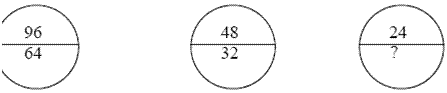(A)     (B)     (C)     (D)     (E)    (1)    (2)    (3)    (4)    (5)
(a) 1
(b) 2
(c) 3
(d) 4
(e) 5
Ans. (c)

Question: Select a figure from amongst the Answer Figures which will continue the same series as established by the five Problem Figures.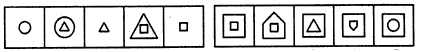(A)     (B)     (C)     (D)     (E)    (1)    (2)    (3)    (4)    (5)
(a) 1
(b) 2
(c) 3
(d) 4
(e) 5
Ans. (d)

Question: Select a figure from amongst the Answer Figures which will continue the same series as established by the five Problem Figures.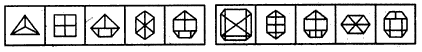(A)     (B)     (C)     (D)     (E)    (1)    (2)    (3)    (4)    (5)

(a) 1
(b) 2
(c) 3
(d) 4
(e) 5
Ans. (e)

Question: Select a figure from amongst the Answer Figures which will continue the same series as established by the five Problem Figures.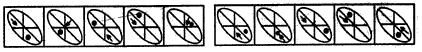(A)     (B)     (C)     (D)     (E)    (1)    (2)    (3)    (4)    (5)

(a) 1
(b) 2
(c) 3
(d) 4
(e) 5
Ans. (a)

Question: Select a figure from amongst the Answer Figures which will continue the same series as established by the five Problem Figures.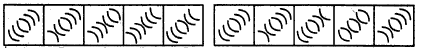(A)     (B)     (C)     (D)     (E)    (1)    (2)    (3)    (4)    (5)

(a) 1
(b) 2
(c) 3
(d) 4
(e) 5
Ans. (c)

Direction for next 3 questions: Each of the following questions consists of two sets of figures. Figures A, B, C and D constitute the Problem Set while figures 1, 2, 3, 4 and 5 constitute the Answer Set. There is a definite relationship between figures A and B. Establish a similar relationship between figures C and D by selecting a suitable figure from the Answer Set that would replace the question mark (?) in fig. (D).

Question: Select a suitable figure from the Answer Figures that would replace the question mark (?).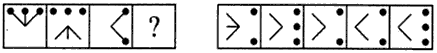(A)       (B)    (C)     (D)              (1)    (2)     (3)    (4)    (5)

(a) 1
(b) 2
(c) 3
(d) 4
(e) 5
Ans. (c)

Question: Select a suitable figure from the Answer Figures that would replace the question mark (?).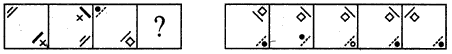(A)       (B)    (C)     (D)              (1)    (2)     (3)    (4)    (5)

(a) 1
(b) 2
(c) 3
(d) 4
(e) 5
Ans. (b)

Question: Select a suitable figure from the Answer Figures that would replace the question mark (?).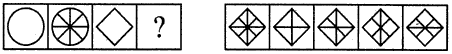(A)       (B)    (C)     (D)              (1)    (2)     (3)    (4)    (5)

(a) 1
(b) 2
(c) 3
(d) 4
(e) 5
Ans. (a)

Related: top Boarding Schools in Ooty

Direction for next 5questions: In each of the following questions you are given a combination of alphabets and/or numbers followed by four alternatives (1), (2), (3) and (4). Choose the alternative which is closely resembles the mirror image of the given combination.

Question: Choose the alternative which is closely resembles the mirror image of the given combination.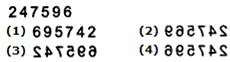(a) 1
(b) 2
(c) 3
(d) 4
Ans. (d)

Question: Choose the alternative which is closely resembles the mirror image of the given combination.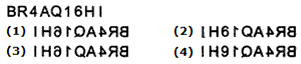(a) 1
(b) 2
(c) 3
(d) 4
Ans. (a)

Question: Choose the alternative which is closely resembles the mirror image of the given combination.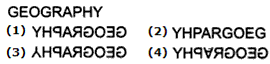(a) 1
(b) 2
(c) 3
(d) 4
Ans. (a)

Question: Choose the alternative which is closely resembles the mirror image of the given combination.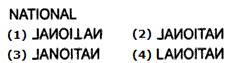(a) 1
(b) 2
(c) 3
(d) 4
Ans. (b)

Question: Choose the alternative which is closely resembles the mirror image of the given combination.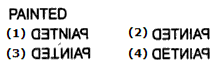(a) 1
(b) 2
(c) 3
(d) 4
Ans. (b)

Direction for next 5 questions: From amongst the figures marked (1), (2), (3) and (4), select the figure which satisfies the same conditions of placement of the dots as in figure (X).

Question: Select the figure which satisfies the same conditions of placement of the dots as in Figure-X.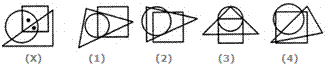(a) 1
(b) 2
(c) 3
(d) 4
Ans. (d)

Question: Select the figure which satisfies the same conditions of placement of the dots as in Figure-X.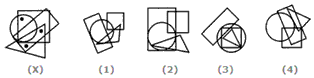(a) 1
(b) 2
(c) 3
(d) 4
Ans. (d)

Question: Select the figure which satisfies the same conditions of placement of the dots as in Figure-X.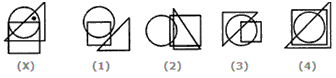(a) 1
(b) 2
(c) 3
(d) 4
Ans. (c)

Question: Select the figure which satisfies the same conditions of placement of the dots as in Figure-X.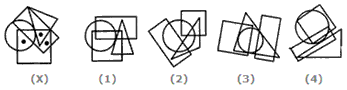(a) 1
(b) 2
(c) 3
(d) 4
Ans. (a)

Question: Select the figure which satisfies the same conditions of placement of the dots as in Figure-X.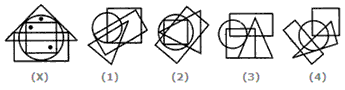(a) 1
(b) 2
(c) 3
(d) 4
Ans. (b)

Question: A clock shows 30 minutes past 2, when both the hands of the clock are rotated by 90° in clockwise direction. What time will the clock show ?
(a) 04 : 45
(b) 05 : 45
(c) 11 : 15
(d) 11 : 16
Ans. (b)

Question: A watch, which gains uniformly, is 3 minutes slow at noon on Monday and is 3 minutes 48 seconds fast at 2 p.m. on the following Monday. What time it was correct ?
(a) 2 p.m. On Tuesday
(b) 2 p.m. On Wednesday
(c) 3 p.m. On Thursday
(d) 1 p.m. On Friday
Ans. (c)

Related: Train coaches in india

Question: How many times are the hands of a clocks coincide in a day ?
(a) 10
(b) 11
(c) 12
(d) 22
Ans. (d)

Question: Two positions of a dice are shown below. Which number will appear on the face opposite to the face with the number 5?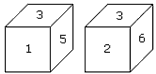(a) 2/6
(b) 2
(c) 6
(d) 4
Ans. (c)

Question: How many points will be on the face opposite to in face which contains 2 points?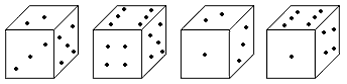(a) 1
(b) 5
(c) 4
(d) 6
Ans. (d)

Question: Which number is on the face opposite to 6?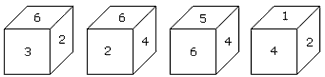(a) 4
(b) 1
(c) 2
(d) 3
Ans. (b)

Question: Two positions of a dice are shown below. When number ‘1’ is on the top. What number will be at the bottom?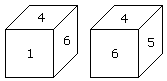(a) 3
(b) 5
(c) 2
(d) 6
Ans. (b)

Question: Two positions of a cube with its surfaces numbered are shown below. When the surface 4 touch the bottom, what surface will be on the top?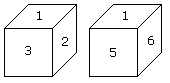(a) 1
(b) 2
(c) 5
(d) 6
Ans. (a)

Direction for next 2 questions: In each of the following questions, four groups of letters are given, three of them are alike in a certain way while one is different. Choose the odd one.

Question: (a) RNJ
(b) XTP
(c) MIE
(d) ZWR
Ans. (d)

Question: (a) PUT
(b) END
(c) OWL
(d) ARM
Ans. (a)

Direction for next 3 questions: In each of the following questions, five (or four) numbers are given. Out of these four (or three) are alike in some way but rest one is different. Choose the one which is different from the other.

Question: (a) 147
(b) 579
(c) 135
(d) 257
Ans. (d)

Question: (a) 2384
(b) 1592
(c) 3756
(d) 3629
Ans. (d)

Question: (a) 3759
(b) 2936
(c) 6927
(d) 4836
Ans. (b)

Directions for next 5 questions: Choose the best alternative

Question:
6 : 18 :: 4 : ?
(a) 2
(b) 6
(c) 8
(d) 16
Ans. (c)

Question: 22.25 : 37 :: 49 : ?
(a) 41
(b) 56
(c) 60
(d) 65
Ans. (d)

Question:
583 : 293 :: 488 : ?
(a) 291
(b) 378
(c) 487
(d) 581
Ans. (b)

Question: 8 : 81 :: 64 : ?
(a) 125
(b) 137
(c) 525
(d) 625
Ans. (d)

Question: 8 : 28 :: 27 : ?
(a) 8
(b) 28
(c) 64
(d) 65
Ans. (d)

Question: Ankit, Bansi, Rohan and Sohan are friends. They play cards. Ankit and Bansi become partners. Sohan faces North. If Ankit faces towards West, then who faces towards South?
(a) Bansi
(b) Rohan
(c) Sohan
Ans. (b)

Question: P, Q, R and S are playing carrom game. P, R and S, Q are partners. S is to the right of R who is facing West. What direction is Q facing then?
(a) North
(b) South
(c) East
(d) West
Ans. (a)

Question: After walking 6 kms, I turned right and covered a distance of 2 kms then turned left and covered a distance of 10 kms. In the end I was moving towards the North. From which direction had I started my journey?
(a) North
(b) South
(c) East
(d) West
Ans. (a)

Question: If ‘South-east’ is called ‘East’, ‘North-west’ is called ‘West’, ‘South-west’ is called ‘South’ and so on. What will ‘North’ be called?
(a) East
(b) North-East
(c) North-West
(d) South
Ans. (c)

Question: A watch reads 9:00 o’clock and I found that the hour hand is pointing South-east. In what direction, the minute hand of my watch is at that time?
(a) East
(b) North
(c) South
(d) North-East
Ans. (c)

Direction for next 3 questions: A, B, C, D, E, & F are related to each other as given here. B is F’s daughter-in-law. D is A’s only grand child. C is D’s only uncle. A has two children F and C, one male & one female (not necessarily in the same order). E is the Father of C.

Question: Who is the grand mother of D ?
(a) B
(b) A
(c) C
(d) D
Ans. (b)

Question: Who is the mother-in-law of B ?
(a) C
(b) D
(c) E
(d) F
Ans. (d)

Question: If a girl G is married into the family, what is the relationship between G and D ?
(a) Mother
(b) Aunt
(c) Mother-in-law
(d) Grand Mother
Ans. (b)

Direction for next 5 questions: In a school, there were five teachers. A and B were teaching Hindi and English, C and B were teaching English and Geography. D and A were teaching Mathematics and Hindi. E and B were teaching History and French.

Question: Who among the teachers were teaching maximum number of subjects?
(a) A
(b) B
(c) C
(d) D
Ans. (b)

Question: Which of the following pairs was teaching both Geography and Hindi?
(a) A and B
(b) B and C
(c) C and A
(d) None of these
Ans. (d)

Question: More than two teachers were teaching which subject?
(a) History
(b) Hindi
(c) French
(d) Geography
Ans. (b)

Question: In a group of cows and hens, the number of legs are 14 more than twice the number of heads. How many cows are in the group?
(a) 5
(b) 7
(c) 11
(d) 12
Ans. (b)

Question: In a chess tournament each of the seven players will play every other player exactly once. How many matches will be played during the tournament?
(a) 12
(b) 21
(c) 14
(d) 13
Ans. (b)

Related: Various banks in India

Direction for next 3 questions: Assume the following :

‘A @ B’ means ‘A is greater than B’ ;
‘A • B’ means `A is either greater than or equal to B’;
`A \$ B’ means `A is equal to B’ ;
‘A B’ means `A is smaller than B’;
`A # B’ means ‘A is either smaller than or equal to B’;
In each question, two statements followed by two conclusions I and II are given. Assuming the statement to be true, state which of the conclusions I and II is / are definitely true ?

Give answer (a) if only conclusion I is true; (b) if only conclusion II is true; (c) if either I or II is true; and (d) if both I and II are true.

Question:
Statements :   P # Q, M • N \$ P
Conclusions:  I. M @ P
II. N # Q
Ans. (d)

Question:
Statements :    L • M, R • T \$ L
Conclusions :  I. T • M
II. R @ L
Ans. (b)

Question:
Statements :    X @ Y @ Z, U @ Z \$ V
Conclusions :  I. V • U
II. X @ V
Ans. (a)

Direction for next 6 questions: Find the missing character in the following questions.

Question:

 5 3 16 9 5 46 7 8 ?

(a) 16
(b) 56
(c) 55
(d) 57
Ans. (d)

Question:

 11 3 49 5 19 ? 7 13 100

(a) 96
(b) 120
(c) 144
(d) 169
Ans. (c)

Question:

 3 6 9 5 8 20 4 7 ?

(a) 11
(b) 14
(c) 28
(d) 12
Ans. (b)

Question:

 3 15 4 7 38 5 3 ? 5

(a) 15
(b) 16
(c) 17
(d) 18
Ans. (d)

Question:

 1 7 9 2 14 ? 3 105 117

(a) 26
(b) 16
(c) 20
(d) 12
Ans. (d)

Question:

 5 26 1 9 84 3 11 ? 5

(a) 146
(b) 116
(c) 126
(d) 136
Ans. (c)

Question:

 12 47 21 10 52 4 64 ? 24

(a) 16
(b) 40
(c) 62
(d) 83
Ans. (d)

Direction for next 9 questions: In each of the following questions, a group of letters is given which are numbered 1, 2, 3, 4, 5 and 6. Below are given four alternatives containing combinations of these numbers. Select that combination of numbers so that letters arranged accordingly form a meaningful word.

Question
I   N   L   A   S   G
1   2   3  4    5   6
(a) 6, 1, 3, 5, 4, 2
(b) 5, 1, 6, 2, 4, 3
(c) 3, 4, 6, 1, 2, 5
(d) 2, 4, 3, 6, 1, 5
Ans. (b)

Question:
T   L   P   N   A   E
1   2   3    4  5   6
(a) 3, 2, 5, 4, 6, 1
(b) 3, 2, 5, 4, 1, 6
(c) 4, 5, 3, 6, 2, 1
(d) 4, 6, 1, 3, 5, 2
Ans. (a)

Question:
I   P   E   L   O   C
1  2   3   4   5    6
(a) 1, 4, 3, 5, 2, 6
(b) 2, 5, 4, 1, 6, 3
(c) 3, 4, 5, 1, 2, 6
(d) 4, 5, 1, 2, 3, 6
Ans. (b)

Question:
R   M   N   B   U   E
1    2    3    4    5   6
(a) 2, 6, 3, 4, 1, 5
(b) 4, 6, 3, 2, 1, 5
(c) 3, 5, 2, 4, 6, 1
(d) 1, 5, 4, 2, 6, 3
Ans. (c)

Question:
T   N   D   R   A   E
1   2   3     4    5   6
(a) 1, 6, 2, 3, 5, 4
(b) 3, 6, 2, 4, 5, 1
(c) 5, 4, 3, 6, 2, 1
(d) 4, 5, 3, 6, 2, 1
Ans. (c)

Question:
E   H   R   A   S   P
1   2   3    4    5    6
(a) 5, 2, 4, 6, 1, 3
(b) 6, 2, 3, 4, 5, 1
(c) 2, 4, 6, 1, 3, 5
(d) 3, 4, 2, 1, 6, 5
Ans. (b)

Question:
T   E   L   S   C   A
1   2   3   4    5   6
(a) l, 2, 3, 4, 6, 5
(b) 4, 6, 5, 1, 2, 3
(c) 5, 6, 4, 1, 3, 2
(d) 6, 5, 3, 2, 4, 1
Ans. (c)

Question:
E   O   C   D   L   I
1    2   3   4   5    6
(a) 3, 2, 5, 4, 6, 1
(b) 4, 2, 3, 5, 6, 1
(c) 3, 2, 4, 5, 6, 1
(d) 4, 2, 3, 6, 5, 1
Ans. (d)

Question:
A   M   D   E   N   R
1    2   3    4   5     6
(a) 2, 1, 3, 5, 4, 6
(b) 6, 4, 2, 1, 5, 3
(c) 3, 4, 5, 2, 1, 6
(d) 1, 6, 2, 4, 5, 3
Ans. (b)

Related: What is a self cheque?

Direction for next 5 questions: In each of the following questions, you are given a combination of alphabets and/or numbers followed by four alternatives (1), (2), (3) and (4). Choose the alternative which is closely resembles the water-image of the given combination.

Question: Choose the alternative which is closely resembles the water-image of the given combination.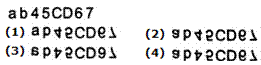(a) 1
(b) 2
(c) 3
(d) 4
Ans. (b)

Question: Choose the alternative which is closely resembles the water-image of the given combination.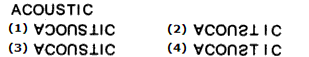(a) 1
(b) 2
(c) 3
(d) 4
Ans. (b)

Question: Choose the alternative which is closely resembles the water-image of the given combination.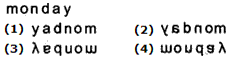(a) 1
(b) 2
(c) 3
(d) 4
Ans. (d)

Question: Choose the alternative which is closely resembles the water-image of the given combination.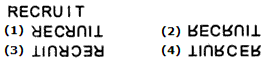(a) 1
(b) 2
(c) 3
(d) 4
Ans. (b)

Question: Choose the alternative which is closely resembles the water-image of the given combination.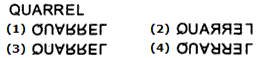(a) 1
(b) 2
(c) 3
(d) 4
Ans. (a)

Direction for next 5 questions : Select the lettered pair that has the same relationship as the given pair of words.

Question: Knife : Chopper :: ? : ?
(a) Walking : Fitness
(b) Swim : Float
(c) Scissors : Cloth
(d) Quilt : Blanket
Ans. (d)

Question:
Triangle : Hexagon :: ? : ?
(a) Cone : Sphere
(b) Rectangle : Octagon
(c) Pentagon : Heptagon
Ans. (b)

Question: Chair : Carpenter
(a) Grass : Gardener
(b) Medicine : Doctor
(c) Bridge : Engineer
Ans. (c)

Question: Fury : Ire :: ? : ?
(a) Amusement : Happiness
(b) Joke : Laugh
(c) Cry : Hurl
(d) Convulsion : Spasm
Ans. (d)

Question:
Food : Hungry :: ? : ?
(a) Thought : Politics
(b) Water : River
(c) Rest : Weary
(d) Wine : Intoxication
Ans. (c)